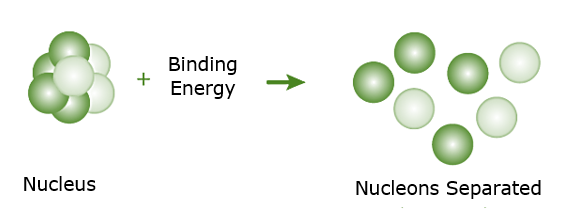GFG App
Open AppBrowser
Continue

# Nuclear Binding Energy – Definition, Formula, Examples

During the twentieth century, Albert Einstein, a well-known scientist, developed the revolutionary theory known as “the theory of relativity.” Mass and energy are interchangeable, according to the theory; mass can be turned into energy and vice versa. This additional dimension to physics benefited in resolving many unsolved problems and provided a platform for many new hypotheses. The presence of Nuclear Binding Energy is one of them. As a result, there is a comprehensive understanding of nuclear mass and inter-nuclei interactions.

The forces that bind nucleons together in an atomic nucleus are considerably stronger than the electrostatic forces that hold an electron to an atom. The relative diameters of the atomic nucleus and the atom (1015 and 1010 m, respectively) demonstrate this. As a result, the energy required to pry a nucleon from its nucleus is substantially more than that required to remove (or ionize) an electron from an atom. All nuclear reactions, in general, involve substantial amounts of energy per particle involved in the reaction. This has a wide range of applications.Now, Let’s understand the concept of Binding Energy in depth!

### What is Nuclear Binding Energy?

Nuclear binding energy is the amount of energy required to keep the protons and neutrons in an atom’s nucleus together. As a result of the energy required to maintain neutrons and protons together, the nucleus remains intact.

It can alternatively be described as the amount of energy required to separate the nucleons. Nuclear binding energy is a measure of how tightly nucleons are bonded to one another. This can be concluded if neutrons and protons are strongly or loosely connected to each other by determining their value. And this can be interpreted as:

• If the nuclear binding energy is large, it takes a lot of energy to separate the nucleons, indicating that the nucleus is very stable.
• If the nuclear binding energy is low, it takes less amount of energy to separate the nucleons, indicating that the nucleus isn’t completely stable.

### Mass Defect and Binding Energy

An atom is made up of a nucleus in the centre and electrons orbiting around it in an orbital pattern. Protons and Neutrons combine to form nuclei, which are referred to as nucleons. As a result, we expect the nucleus’ mass to be equal to the sum of the individual masses of neutrons and protons. However, this is not the case. The nucleus’ overall mass (mnuc) is smaller than the sum of the individual masses of neutrons and protons that make it up. This disparity in mass is referred to as a mass defect.

Δm = Zmp+(A−Z)mn−mnuc

where,

Zmp is the total mass of the protons.

(A-Z)mn is the total mass of the neutrons.

mnuc is the mass of the nucleus.

The mass energy is equivalent, according to Einstein’s theory of relativity. That is, the total energy of a system is measured by its mass. E=mc2 is a well-known equation. As a result, the nucleus’ overall energy is smaller than the sum of the energies of individual protons and neutrons (nucleons). This means that when the nucleus breaks down into its constituent nucleons, some energy is released in the form of heat energy. (This is an exothermic process.) The energy emitted here is expressed mathematically as

E = (Δm)c2

Consider the case of a nucleus that has been broken. A specific quantity of energy is placed into the system to do this. Nuclear binding energy is the amount of energy necessary to do this. As a result, nuclear binding energy can be defined as, The minimum energy required to separate nucleons into its constituent protons and neutrons. and is given by-

Eb = (Δm)c2

Hence, the difference in the mass is converted into Nuclear binding energy.

### Binding Energy per Nucleon (BEN)

The binding energy per nucleon (BEN), which is defined by,

BEN = Eb / A

It is one of the most important experimental quantities in nuclear physics.

This is the average energy necessary to remove a single nucleon from a nucleus, which is comparable to the ionization energy of an electron in an atom. The nucleus is relatively stable if the BEN is reasonably large. Nuclear scattering tests are used to calculate BEN values.

### Sample Problems

Problem 1: What is Mass Defect?

Solution:

The difference between the expected and actual mass of an atom’s nucleus is known as a mass defect. This discrepancy is due to the binding energy of a system manifesting as more mass.

Problem 2: Determine the Binding energy per Nucleon for a 4020Ca nucleus.

Solution:

The Total Binding energy of 4020Ca nucleus.

= 20mp + 20 mn – M ( 4020Ca)

= 20 × 1.007825 + 20 × 1.008665 – 39.962589.

= 0.367211 u = 0.367211 × 931 MeV = 341.87 Mev.

∴ Binding energy per nucleus = 341.87/40 MeV/nucleon.

= 8.55 MeV/nucleon

Problem 3: Determine the  binding energy of a nitrogen nucleus

Solution:

The nucleus of (147N) nitrogen it contains 7 protons and 7 neutrons.

mp = 1.00783 u

Mass of Neutron, mn = 1.00867

In 147N there are 7 protons and 7 neutrons,

Here, m = 14.00307 u

∴ Mass Defect, ∆m = (7mp + 7 mn) – m

= 7 × 1.00783 u + 7 × 1.00867 – 14.00307 u

= 0.11243 u

Binding energy of nucleus,

∆m × 931 MeV = 0.11243 x 931 MeV = 104.67 MeV

Problem 4: How to find the binding energy?

Solution:

The binding energy is determined by the formula:

Binding Energy = mass defect x c2

where c= speed of light in vacuum

Problem 5: Give the expression for Mass defect.

Solution:

Δm=Zmp+(A−Z)mn−mnuc

where,

Zmp is the total mass of the protons.

(A-Z)mn is the total mass of the neutrons.

mnuc is the mass of the nucleus.

My Personal Notes arrow_drop_up